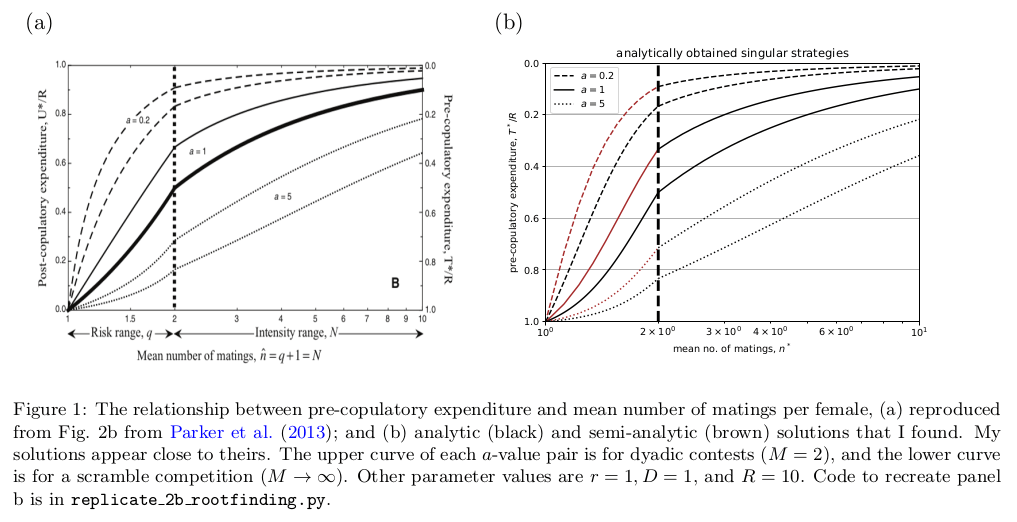# New solutions for Parker sperm competition model

Parker et al. (2013) created a general model for sperm allocation under a trade-off between male investment of resources $$R$$ into pre-copulatory effort (e.g. search time) $$T$$ versus post-copulatory effort (e.g. ejaculate) $$U$$. Their model is interesting because it encompasses a range of different scenarios of female remating and the type of competition between males. For female remating scenarios, the risk model has females mating a second time with probability $$q$$, with the possibility that being first or second leads to higher fertilisation rate (`loaded raffles’); whereas the intensity model has females mating with $$N > 2$$ males on average. For male competition scenarios, scramble competition has every male competing for every mating opportunity ($$M \rightarrow \infty$$); alternatively, groups of $$M$$ males may compete per mating opportunity. The average number of matings $$n$$ that a mutant male achieves was given a nice flexible functional form (their Eq. 6), so that the shape of the relationship between a mutant’s relative number of matings versus pre-copulatory expenditure (their Fig. 1) could be controlled by a parameter $$a$$.

Parker et al. (2013) used these models to obtain the ESS for the units of sperm per ejaculate, $$s$$. They were able to solve the ESS analytically for the following scenarios: $$a=1$$, implying that males gain matings with increased pre-copulatory expenditure in a linear way; and $$M \rightarrow \infty$$, implying scramble competition. For other scenarios, there was no analytical solution, basically because the flexible form they gave $$n$$.

Out of curiosity, I rewrote their model so that the pre-copulatory effort $$T$$ was treated as the trait under selection. I found that, by doing so, I was able to get analytic solutions for three of their four scenarios for the full $$a$$ and $$M$$ parameter range, and a semi-analytic solution for the remaining scenario. Python code to reproduce my results can be found on Github.

When $$T$$ is the trait space, their Eq. 6 (the number of matings the mutant male obtains) can be left as it is:
$n(M,T,\hat{T}) = M \hat{n} \left( \frac{T^a}{T^a + (M-1)\hat{T}^a} \right).$
This avoids the complication introduced by having $$n$$ in terms of $$s$$ in their Eq. 9. Then the derivative $$d n(M,T,\hat{T}) / dT$$ can be obtained straightforwardly.

To use $$T$$ as the trait space, the expressions for the value for each mating, $$v(s,\hat{s})$$, must be converted into $$v(T,\hat{T})$$. The conversion is obtained by using their Eq. 3a
$s = \frac{R – T} {n(T,\hat{T})D},$
and
$\hat{s} = \frac{R – \hat{T}} {\hat{n}},$

They give the expression for fitness
$w(T, \hat{T}) = n(T, \hat{T}) \: v(T, \hat{T}).$
Then the singular strategies can be found by solving for $$T^*$$ such that
$\left. \frac{d w(T,\hat{T})}{dT} \right|_{T = \hat{T} = T^*} = 0$

For the intensity model, I found two singular strategies, $$T^*=R$$, and
$T_{I}^* = \frac{{\left(M – 1\right)} R a}{{\left(M – 1\right)} a + M \hat{n} – M}$

For $$M \rightarrow \infty$$ (taking limit of above)
$T_{I}^* = \frac{ R a}{ a + \hat{n} – 1}$

For the risk model, a general analytic expression for $$T^*$$ cannot be found. Instead, a semi-analytic solution is found, where the roots of an equation must be solved with a numerical root-finding algorithm. (I haven’t included the equation here because it’s a bit long, but you’ll find it in the code).

For $$M \rightarrow \infty$$, two singular strategies are obtained, $$T^*=R$$, and
$T_{R}^* = \frac{{\left({\left(\hat{n}^{2} – 3 \, \hat{n} + 3\right)} r^{2} – 2 \, {\left(\hat{n} – 2\right)} r + 1\right)} R a}{{\left(a \hat{n}^{2} – 3 \, a \hat{n} + 3 \, a\right)} r^{2} – {\left({\left(2 \, a + 1\right)} \hat{n} – \hat{n}^{2} – 4 \, a\right)} r + a}.$

To check that my solutions above were correct, I tried to replicate their Fig. 2b. My solutions appear to be pretty close their results.#### Reference:

Parker, G. A., Lessells, C. M. and Simmons, L. W. (2013). Sperm competition games: a general model for precopulatory male–male competition, Evolution 67(1): 95–109.